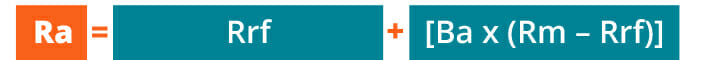# Portfolio Tools: Capital Asset Pricing Model

The Capital Asset Pricing Model (CAPM) is a model that describes the relationship between expected return and risk of investing in a security. It shows that the expected return on a security is equal to the risk-free return plus a risk premium, which is based on the beta of that security. Below is an illustration of the CAPM concept.

### CAPM Formula and Calculation

CAPM is calculated according to the following formula:Where:

Ra = Expected return on a security
Rrf = Risk-free rate
Ba = Beta of the security
Rm = Expected return of the market

Note: “Risk Premium” = (Rm – Rrf)

The CAPM formula is used to calculate the expected return on investable asset. It is based on the premise that investors have assumptions of systematic risk (also known as market risk or non-diversifiable risk) and need to be compensated for it in the form of a risk premium – an amount of market return greater than the risk-free rate. By investing in a security, investors want a higher return for taking on additional risk.

### Expected Return

The “Ra” notation above represents the expected return of a capital asset over time, given all of the other variables in the equation.  The expected return is a long-term assumption about how an investment will play out over its entire life.

### Risk-Free Rate

The “Rrf” notation is for the risk-free rate, which is typically equal to the yield on a 10-year US government bond.  The risk-free rate should correspond to the country where the investment is being made, and the maturity of the bond should match the time horizon of the investment.  Professional convention, however, is to typically use the 10-year rate no matter what, because it’s the most heavily quoted and most liquid bond.

The beta (denoted as “Ba” in the CAPM formula) is a measure of a stock’s risk (volatility of returns) reflected by measuring the fluctuation of its price changes relative to the overall market. In other words, it is the stock’s sensitivity to market risk. For instance, if a company’s beta is equal to 1.5 the security has 150% of the volatility of returns of the market average. However, if the beta is equal to 1, the expected return on a security is equal to the average market return.  A beta of -1 means security has a perfect negative correlation with the market.